# A Single-Input Dual-Output DC-DC Converter for Powertrain of PEM Fuel Cell Vehicle

A Single-Input Dual-Output DC-DC Converter for Powertrain of PEM Fuel Cell Vehicle

Mudadla Dhananjaya Devendra PotnuruB. Krishna Chaitnya NareshPatnana Jagadish Kumar Bokam

Dept. of EEE, Anil Neerukonda Institute of Technology & Sciences, Visakhapatnam, AP, India

Dept. of EEE, Gayatri Vidya Parishad College of Engg. for women, Visakhapatnam, AP, India

Dept. of EEE, CBIT Engineering College, Hyderabad, India

Dept. of EEE, GITAM University, Visakhapatnam, AP, India

Corresponding Author Email:
devendra.p.07@gmail.com
Page:
162-171
|
DOI:
https://doi.org/10.14447/jnmes.v25i3.a02
11 February 2022
|
Accepted:
20 June 2022
|
Published:
31 August 2022
| Citation

OPEN ACCESS

Abstract:

Multi-output converter plays a vital role in portable electronic and electric vehicle (EV) applications. In this regard, a new single-input dual output (SIDO) converter is proposed in this paper. Most of the single-input dual-output converter configurations presented by various researchers in the domain of multi-output converters function under particular assumptions about operational duty cycle and inductor current.Also, the issue of cross- regulation is still prevalent while operating the loads in many SIDO converters.The proposed configuration generates two output voltages in boost and buck-boost modes without any constraints on the duty ratioor inductor currents. In addition, it doesn't encounter cross-regulation problems; subsequently, the output voltage V01 (V02) is not influenced by load changes in i02 (i01). To verify the feasibility and effectiveness of the proposed configuration, a 200 W prototype circuit is developed; simulation and experimental results are validated.

Keywords:

Fuel cell EVs, PEM Fuel cell, multiport converters.

1. Introduction

Multi-input and multi-output converters, also called Multiport converters (MPCs), drew the attention of researchers, specifically working in the areas of grid- connected independent energy sources portable electronic and electric vehicle (EV) applications. Moreover, MPCs involve less number of components and deliver a compact structure compared to various DC-DC converters,.As a result, the system's complexity and cost decrease, and the power density increases.Thus MPCs are an excellent solution for grid- connected systems, electric vehicles, and portable electronic applications[3-7].

As proposed in  and , a new SIMO converter simultaneously generates boost, buck, and inverted outputs controlled independently. However, producing ‘n’ voltage levels require n + 2 switches, which increases the size and cost. The state-space equations and output voltages in  have been further modified and rectified in . The single coupled inductor-based SIMO buck is presented in  with lesser output inductor current ripple than single inductor SIMO converters. Gayatri et al. elaborately explained the comparative performance of SIDO converters and discussed the cross-coupling issues with coupled inductors . And also proposed that the coupled inductor SIDO converter has a better steady-state and transient performance. However, in a SIMO configuration with a single inductor, the inductor is changed between the outputs, resulting in significant ripples and cross-regulation issues.

Different control approaches are proposed in the literature to overcome the cross-regulation issue in a single inductor-based SIMO converter; a current predictor is proposed in  instead of the conventional charge-balance approach. However, the control approach in  is somewhat complicated to generate the duty ratios for active switches. Similarly, the deadbeat- based control approach is presented in . It is based on output current observer, and hence it is sensitive to the noise and /or significant parametric variations. In , a multivariable digital controller-based SIMO converter is proposed to minimize the voltage ripples, suppress the cross- regulation problems, and regulate the output voltages. However, controller design may lead to an increase in the complexity of the converter.

A non-isolated and single switch SIMO converter topology is presented in . It has a low device count with hence low cost and smaller size. However, it may be challenging to regulate the outputs independently with different duty ratios. Non-isolated SIMO converters [16-24] were also proposed to tackle the problems in a single inductor SIMO converter that independently regulates the output voltages. In , a new SIDO converter topology is presented with buck and super lift converter integration for generating the step-up and step-down output voltages for electrical vehicle applications. It has a constraint on-duty ratio viz. D2 <D1. The presented topologies in [17-18] could independently regulate the output voltage, and even the number of semiconductor devices required is less. However, the duty ratio selection is such that iL1 > iL2 and outputs are arbitrary. Another SIMO converter, a combination of high gain step-up and Sepic converter, is suggested for PV applications in . The output voltages are higher and improved than the input voltage in this configuration using capacitors and diodes. However, with additional capacitors and diodes, the overall cost and conduction losses also increase. A new SIDO buck-boost converter is developed to generate the same positive and negative output voltage . A topology synthesis process has been developed in  to develop various combinations.

A three output converter is suggested in  with high voltage gains, but it has a reduced number of power IGBTs than similar configurations. The conduction losses are higher due to more number of diodes. In , a novel SIMO converter structure is introduced.It uses a reduced passive filter element size and has a low magnitude of voltage stress on devices. Nevertheless, it has more device count, affecting the cost and size. High-density multi-output converter is proposed in  for portable applications. This configuration has a front-end switched-capacitor which improves the power density and limits the switching losses. Most recently, researchers proposed Maximum Power Point Tracking (MPPT) for Proton Exchange Membrane (PEM) Fuel cellbased DC-DC converters-. In , MPPT using hybrid artificial bee colony algorithm with a fuzzy controller is developed for boost converter, ANN is suggested in , for an high step up and quadratic boost converters and in , a PSO based MPPT for buck converter for proton‐exchange membrane fuel cell-based buck converter. PEM Fuel Cell has good application potential in electric vehicles, distributed generation, portable power systems,microgrid and aerospace equipment.

A new SIDO topology is presented in this paper to generate two output voltages withimproved output voltage gains and to regulate the output voltages individually without any control constraints.The main advantage of the circuit configuration is that it avoids cross-regulation issues during the control of loads.The following is how the manuscript is organized: Section 2 describes the proposed SIDO architecture and operation modes. Section 3 discusses small-signal analysis. Section 4 covers the parameter design technique, power loss, and comparison analysis. Application of the proposed converter is presented in section.5. The recommended configuration's performance is verified 6, and the conclusions are provided in Section 7.

2. Proposed SIDO Configuration and Modes of Operation

The structure of the proposed single input dual-output DCDC converter is depicted in Figure 1. This configuration consists of VDC as the input voltage, active switches (S1-S3), diodes (D1 and D2), and passive filter elements (L1-C1) and (L2-C2). It can generate two different output voltages, one as boost voltage (V01) and the other as either buck or boost voltage (V02). Also, this configuration can operate under a switch fault condition. It can generate the boost and buck output voltages with independent duty ratios.

The proposed structure has following advantages:

• The circuit structure is simple with out any operational constraints on the operating duty cycle (D1 > D2 or D2 < D1 or D1 = D2 )
• Further, no control constraint likeiL1 > iL2 or iL1 < iL2
• It can generate the independent output voltages
• The converter one output voltage is the positive buck-boost output which does not require inverting circuit. And another output voltage is boost voltage.
• The proposed configuration operates as SIDO, delivering the boost and buck outputs.

## 1.png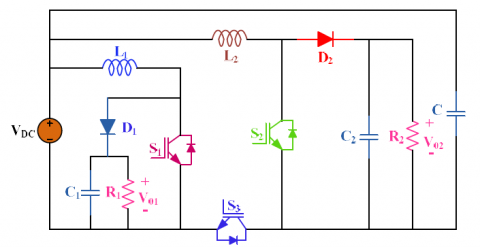Figure 1. Proposed SIDO configuration

The other application areas of the proposed configuration are dc nanogrids, bias supplies, and solar battery chargers.

2.1 Modes of operation

Switching state 1:

The devicesS1, S2, and S3 are controlled in this mode. Figure 2(a) depicts the current flow, and during this mode, inductors are magnetized by the input DC source.Further, the capacitorsare discharged their energy to the loads. The governing equations are given in (1)-(4) during this mode.

$i_{L_1}(t)=\frac{V_{D C}}{L_1} t+i_{L_1(0)}$                     (1)

$v_{C_1}(t)=v_{C_1(0)} e^{\frac{-1}{R_1 C_1} t}$                       (2)

$i_{L_2}(t)=\frac{V_{D C}}{L_2} t+i_{L_2(0)}$                   (3)

$v_{C_2}(t)=v_{C_2(0)} e^{\frac{-1}{R_2 C_2} t}$                     (4)

Switching state 2:

The body diode of the switches S2 and S4 are conducting as shown in Figure 2(b). The equations describing this mode aregiven in (5)-(8),

$i_{L_1}(t)=\frac{V_{D C}}{R_1}+e^{-\alpha_1 t}\left[c_1 \cos \omega_{d 1} t+c_2 \sin \omega_{d 1} t\right]$                      (5)

$v_{C_1}(t)=V_{D C}-\frac{L_1}{2 C_1} e^{-\alpha_1 t}\left[\begin{array}{l}\cos \omega_{d 1} t\left(\frac{c_1}{R_1}-\omega_{d 1} c_2\right)+ \\ \sin \omega_{d 1} t\left(\omega_{d 1} c_1+\frac{c_2}{R_1}\right)\end{array}\right]$                         (6)

$i_{L_2}(t)=e^{-\alpha_2 t}\left[c_3 \cos \omega_{d 2} t+c_4 \sin \omega_{d 2} t\right]$                        (7)

$v_{c_2}(t)=-L_2 e^{-\alpha_2 t}\left[\begin{array}{l}\left(-\alpha_2 c_3+\omega_{d 2} c_4\right) \cos \omega_{d 2} t \\ +\left(\omega_{d 2} c_3-\alpha_2 c_4\right) \sin \omega_{d 2} t\end{array}\right]$                   (8)

## 2.pngFigure 2. Switching states of the converter: (a). state 1 and (b) state 2

where

$\alpha_1=\frac{1}{2 R_1 C_1}, \omega_{d 1}=\frac{1}{2} \sqrt{\left(\frac{1}{R_1^2 C_1^2}-\frac{4}{L_1 C_1}\right)}$,

$\alpha_2=\frac{1}{2 R_2 C_2}$ and $\omega_{d 2}=\frac{1}{2} \sqrt{\left(\frac{1}{R_2^2 C_2^2}-\frac{4}{L_2 C_2}\right)}$

Output voltages of the proposed configuration are as follows

$V_{01}=\frac{V_{D C}}{\left(1-D_1\right)}, V_{02}=V_2+\frac{D_2 V_{D C}}{\left(1-D_2\right)}$                     (9)

where D1 and D2 are the duty ratio of the switches S1, S2, and S3, respectively.

It is observed that during the switching state-1and 2 operation, the load (R1) and (R2) are isolated from the ground, as shown in Figure 3. It is observed that during any mode of operation, all the loads are not connected at one ground point electrically; however, they might be connected physically at a common ground. Moreover, this configuration of the circuit is such that energy associated with the inductor is confined to one output only and is not shared with the other outputs during the control and also independently regulates output voltages with independent duty-cycles. As a result, load current i02 (i01) does not influence the output voltages V01 (or V02). Hence the proposed converter loads are isolated during the control and avoid all the cross-regulation problems. More importantly, the configuration has two independent outputs without control constraints like iL1 >iL2 or D1+D2<=1.

2.2 Semiconductor stress analysis

Semiconductor stresses of the proposed configuration are presented in Eq. in (10)-(21) as follows .

Voltage stresses

$V_{S_1}=V_{01}, V_{D_1}=V_{01}$

$V_{S_2}=V_{S_3}=\left(\frac{V_{D C}+V_{02}}{2}\right)$

$V_{D_2}=\left(V_{D C}+V_{02}\right)$                (10)

Current stresses

Mode 1:

$i_{S_1}=i_{L_1}, i_{S_2}=i_{S_3}=i_{L_2}, i_{D_1}=i_{D_2}=0$              (11)

Mode 2:

$i_{S_1}=i_{S_2}=i_{S_3}=0, i_{D_1}=i_{L_1}, i_{D_2}=i_{L_2}$                (12)

3. Small Signal Modeling

The system transfer functionsfor the developed circuit configuration are derived as procedure given in , and their equations are described in (13)-(28). The equations are written in a state-space form (13)-(14).

$\dot{x}(t)=B.x(t)+C.u(t)$                 (13)

$y(t)=D . x(t)+E . u(t)$                       (14)

The above equations forn are the switching modes in (15) and (16) during one switching period.

$\dot{x}(t)=B_n x(t)+C_n u(t)$                 (15)

$y(t)=D_n x(t)+E_n u(t)$                       (16)

The state equations are followed as,

\begin{aligned} \dot{x}(t)=& d B_1 x(t)+(1-d) B_2 x(t) \\ &+d C_1 u(t)+(1-d) C_2 u(t) \end{aligned}                        (17)

\begin{aligned} y(t)=& d D_1 x(t)+(1-d) D_2 x(t) \\ &+d E_1 u(t)+(1-d) E_2 u(t) \end{aligned}                           (18)

To linearize the above equations, perturbations are considered in all parameters below.

$d=D+\hat{d}$                  (19)

$U(t)=U+\hat{u}(t)$                  (20)

$X(t)=X+\hat{x}(t)$                   (21)

$Y(t)=Y+\hat{y}(t)$                    (22)

The average model of the converter is given in matrix form,

$\frac{d}{d t}\left[\begin{array}{c}i_{L_1}(t) \\ i_{L_2}(t) \\ v_{C_1}(t) \\ v_{C_2}(t)\end{array}\right]=B\left[\begin{array}{c}i_{L_1}(t) \\ i_{L_2}(t) \\ v_{C_1}(t) \\ v_{C_2}(t)\end{array}\right]+C V_{D C}$                   (23)

where

$B=\left[\begin{array}{cccc}0 & 0 & \frac{-\left(1-D_1\right)}{L_1} & 0 \\ 0 & 0 & 0 & \frac{\left(1-D_2\right)}{L_2} \\ \frac{\left(1-D_1\right)}{C_1} & 0 & \frac{-1}{R_1 C_1} & 0 \\ 0 & \frac{\left(1-D_2\right)}{C_2} & 0 & \frac{-1}{R_2 C_2}\end{array}\right]$                        (24)

$C=\left[\begin{array}{c}\frac{1}{L_1} \\ \frac{D_2}{L_2} \\ 0 \\ 0\end{array}\right], \mathrm{D}=\left[\begin{array}{llll}0 & 0 & 1 & 0 \\ 0 & 0 & 0 & 1\end{array}\right]$                        (25)

The output voltages $\hat{v}_{01}$ a $\hat{v}_{02}$ re determined by $\hat{d}_1$ and $\hat{d}_2$, as illustrated

$\hat{v}_{01}(s)=G_{v d 1} \hat{d}_1(s)$

$\hat{v}_{02}(s)=G_{v d 2} \hat{d}_2(s)$                          (26)

The control transfer functionsfor the proposed configuration are as below with a small-signal analysis.

$\frac{\hat{v}_{01}(s)}{\hat{d}_1(s)}=\frac{V_{D C}}{\left(1-D_1\right)\quad^2}\left[\frac{1-s \frac{L_1}{R_1\left(1-D_1\right)\quad^2}}{1+s \frac{L_1}{R_1\left(1-D_1\right)\quad^2}+s^2 \frac{L_1 C_1}{\left(1-D_1\right)\quad^2}}\right]$                     (27)

$\frac{\hat{v}_{02}(s)}{\hat{d}_2(s)}=\frac{V_{D C}}{R_2}\left[\frac{1+s C_2 R_2}{1+s \frac{L_2}{R_2}+s^2 L_2 C_2}\right]$                         (28)

4. Parameters Design and Converter Analysis

4.1 Design of parameters

This converter's parameters design is followed as per the procedure given in .

$L_{1 \min }=\frac{2}{27} \frac{R_{L 1 \max }}{f_s}$

$L_{2 \min }=\frac{R_{L 2 \max }\left(1-D_{\min }\right)}{2 f}$                        (29)

fs = switching frequency, Dmin = Minimum duty cycle

Current ripple through inductors is given in (30)

$\Delta i_{L_1 \max }=\frac{V_{01} D_{\min }\left(1-D_{\min }\right)}{f_s L_1}$

$\Delta i_{L_2 \max }=\frac{V_{02}\left(1-D_{\min }\right)}{f_s L_2}$                         (30)

Calculation of filter capacitance value is

$C_{1 \min }=\frac{D_{\max } V_{01}}{V_{c p p} R_{L 1 \max } f_s}, C_{2 \min }=\frac{D_{\max }}{2 r_c f_s}$                     (31)

where

V01, 02=Output voltage, fs=Switching frequency, Dmax =Max. duty ratio, Dmin = Min. duty ratio Vcpp =Peak-to-peak

$V_{c p p}=\frac{V_r}{2}$                                      (32)

The ripple voltage (Vr) is 1% V0

4.2 Power losses calculations

Power losses are essential for calculating efficiency as follows , and is shown in Eq. (29)-(29).

$P_{\text {loss_IGBT }}=P_{C o n}+P_{S w}$                    (33)

The IGBT conduction losses are

${{P}_{\text{con }}}=\frac{1}{T}\underset{0}{\overset{T}{\mathop{{{}^\backprime{o}}}}}\,\left( R_{On}^{{}}{{i}_{F}}+{{V}_{{{F}_{O}}}} \right){{i}_{F}}dt$                      (34)

Where, Ron= Switch ON-state resistance, VFo= Threshold voltage,iF= Forward current, and T = Switching period=1/f. The switching losses are calculated using the energy associated during ON and OFF.

$P_{s w}=\left(E_{O F F, j}+E_{O N, j}\right) \times f$                              (35)

The efficiency of the proposed converter is

$\eta=\frac{P_{\text {out }}}{P_{\text {out }}+P_{\text {sw }}+P_{\text {con }}}$                                (36)

Table 1. Parameter Specifications

 Parameter Simulations Experiments Input DC source 50 Volts 50 Volts Output magnitude 100 Volts 100 Volts Load currents (I01/I02) 2/1.5 Amp 2/1.5 Amp Switching frequency (f) 50 kHz 50 kHz Inductor L1 0.6 mH 0.5mH Inductor L2 1.5 mH 2 mH Capacitor C1 200uF 220uF Capacitor C2 360 uF 470 uF

4.3 Performance comparison

This subsection presents performance comparison in terms of power components, energy storage elements, maximum stresses on active switches, etc., presented in this subsection with recently proposed MLCs. In Table.1, the details are given. In , it has reduced the power devices. However, it may be challenging to regulate the outputs independently with different duty ratios. In  has less number of components and proposed for EV’s auxiliary power supply application. Nevertheless, it has a constraint of iL1 > iL2 for generating output voltages.

## 3.png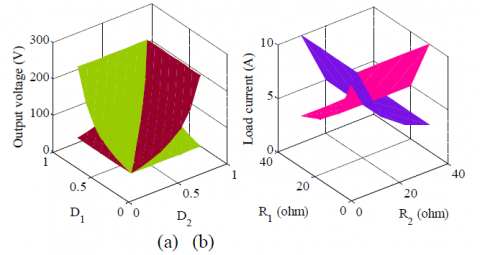Figure 3. (a) Output voltage vs. duty ratio, and (b) load current vs. load

A SIMO converter illustrated in  generates positive and negative output voltages. However, it utilizes more components, increasing the size, cost, and power losses. A new SIDO converter is presented in . It has low semiconductor stress. However, it has more device count, affecting the cost and size. A high-density multioutput converter is suggested in  for portable electronic applications. This configuration has more active switches, which may affect the converter efficiency.

The maximum voltage stress on the power switches of topology given in  and the proposed converter is the same magnitude. It is equal to the sum of input and output voltages. It is equivalent to supply voltage for topology given in  and comparable to output voltage topology presented in . The suggested converter in  has less voltage stress, i.e., equals to half of the output voltage. However, the out voltage magnitude is checked at different duty ratios; the corresponding output voltage vs. duty ratio plot is shown in Figure 3(a). Similarly, load current vs. load is depicted in Figure 3(b). From plots 3(a) and (b), it is observed that without any duty cycle constraint, the converter can generate the different output voltages and also independently regulate the outputs.

From this comparative analysis, it was observed that each circuit configuration has some advantages and some drawbacks. The proposed topology is simple in design, and without any limitations on inductor currents andoperating duty ratio, it can generate two different output voltages, which also allows to regulate them independently. The output voltageV01 is in boost mode, and the other is either buck or boost voltage (V02). Also, this configuration can be extended to operate under a switch fault condition. Moreover, It has low voltage and current stresses.

Table 2: Comparison between different SIMO topologies

## 1.png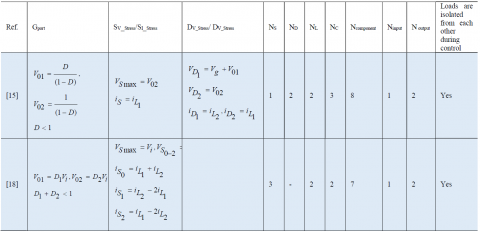## 2.png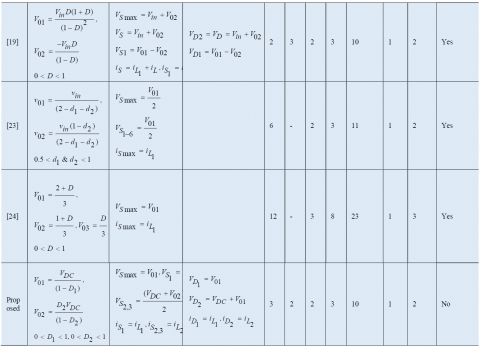5. Application of the Proposed Converter for Fuel Cell Vehicles

The proton exchange membrane fuel cell (PEMFC) can be used to drive the electric vehicle with hydrogen (H2) and oxygen (O2) are input sources. Figure 4(a) depicts the PEMFC's basic structure. The H2 is oxidized as described in (37) at the anode when it operates. Further, the hydrogen will combine with oxygen and produce water (H2O) as described .

## 4a.png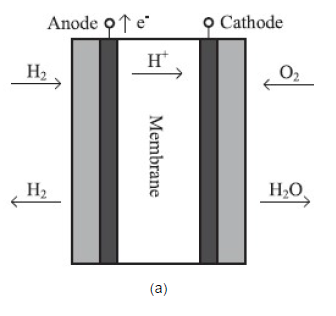## 4b.png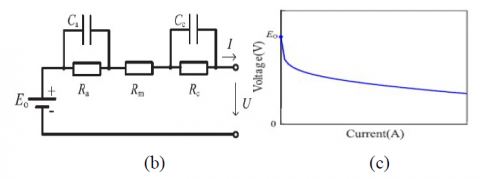Figure 4. (a) Basic structure of PEMFC, (b) Simplified circuit for PEMFC, and (c) Output characteristic curve of voltage-current (V–A) of PEMFC .

$\mathrm{H}_2 \rightarrow 2 \mathrm{H}^{+}+2 e^{-}$                                (37)

$\mathrm{O}_2+4 \mathrm{H}^{+}+4 e^{-} \rightarrow 2 \mathrm{H}_2 \mathrm{O}$                               (38)

The electric charge “e−” will facilitate electric current when the load is connected across anode and cathode. The equivalent circuit is shown in Figure 4(b), where the parameters Ca is capacitance and Ra is the resistor due to anode reaction.The open-circuit voltage is represented with Vo. Similarly, the Cc and Rc are capacitors and resistor parameters due to cathode reactions. The equivalent series resistance of the membrane is expressed with Rm for the PEMFC. Finally, Vt and Iare the output voltage and current of the fuel cell. Therefore, the output voltage Vt can be expressed as

$V_t=V_O-I\left(\mathrm{R}_{\mathrm{a}}+\mathrm{R}_{\mathrm{m}}+\mathrm{R}_{\mathrm{c}}\right)$                         (39)

The output terminal voltage vs. load current characteristics with PEMFC is shown in Figure 5(c). It is observed that output voltage will vary with load variation on the fuel cell and decrease with the increase in the load current.

Application of the proposed SIDOconverter for fuel cell vehicles

The proposed configuration is suitable for fuel cell-based electric vehicles based on its characteristics explained previously. The power train of the fuel cell-based EV is shown in Figure 5, wherein the input power sources consist of fuel cell and supercapacitor or battery pack. The dc-dc converters are required to interface the fuel cell power source and supercapacitor stack with the dc bus and control the power fed to the motor and EV axillaries. This hybrid energy scheme is such that the average power generated by the fuel cell source is used to drive the motor. In contrast, a supercapacitor or battery pack can observe the frequency regenerative power using a bidirectional converter.

Moreover, the common dc bus will also feed the power to the EV auxiliaries like wiper or LED lamps, etc. The characteristic of the fuel cell is such that the terminal output voltage is variable for load variations. Therefore, the power converter with a wide range of output gains is required to meet the characteristics of the fuel cell source.

The proposed DC-DC converter is designed with a wide range of DC voltage gains, and hence it is suitable for fuel cellbased power sources. As shown in Figure 5 with low voltage fuel cell with high voltage dc bus. The converter generates the boost voltages as given in equation (9) for different duty ratios. As the fuel cell has a slow dynamic response and gives the average power to the dc bus, the supercapacitor fed bidirectional converter will be used during the vehicle's acceleration or deceleration (braking). The supercapacitor drives the loads during the acceleration and observes the regenerative power completely during deceleration or braking. The fuel cell source will drive the vehicle steady-state and charge the supercapacitor when needed. The switch fault tolerance feature is also included in the proposed work to improve the reliability.

## 5.png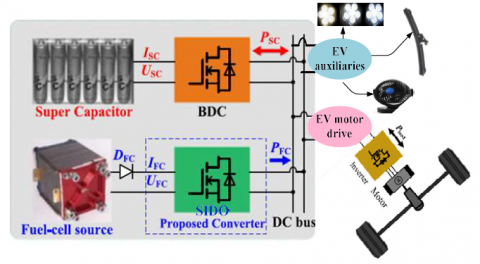Figure 5. Powertrain of fuel cell vehicles with the DC-DC converter

6. Simulation and Experimental Verification

6.1 Simulation verification

The MATLAB/Simulink environment is used to verify the working of the suggested topology with the parameter values described in Table 1. The input voltage is VDC = 50 V, switching frequency of 50 kHz at duty ratio is fixed at 50%. The V01 and V02 are shown in Figure 6(a) and (c), respectively, and corresponding inductor currents iL1 and iL2 are demonstrated in Figure 6(b) and (d), respectively. It is noted that the output voltage nearly equals theoretical values. The transient behavior of the proposed configuration is also checked by suddenly changing load; the related results areshown in Figure 7(a) and (b) for V01 and V02, respectively. The voltage and current stresses are illustrated in 8(a-e) for the active switches at 50 % duty ratio as per areas equations are given in Table.2.

## 6.png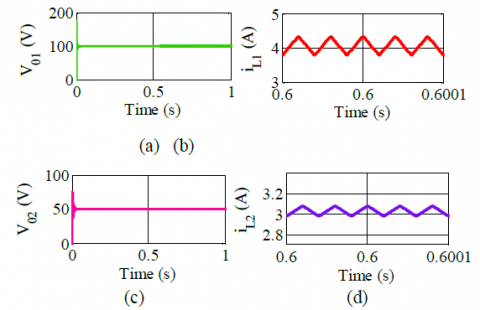Figure 6. (a) V01,(b) iL1,(c) V02and (d) iL2

## 7.png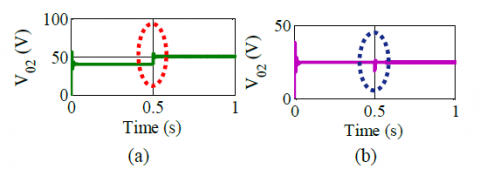Figure 7. Output voltage at load variations: (a) V01 and (b) V02

## 8a.png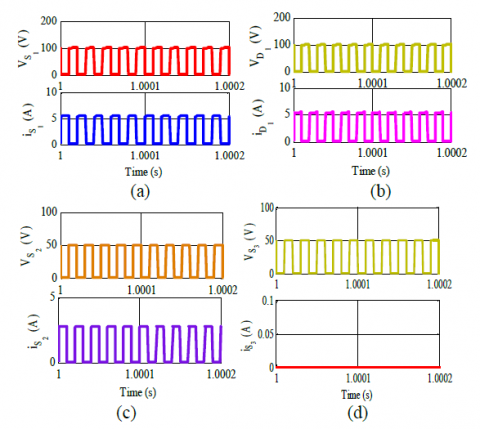## 8b.png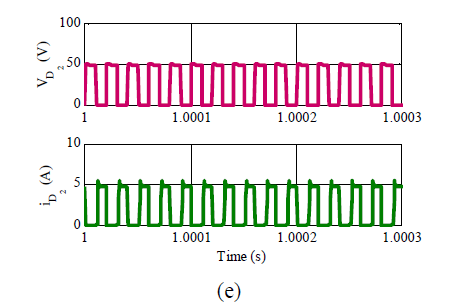Figure 8. Voltage & current stresses on: (a) S1, (b) D1, (c) S2 (d) S3, and (e) D2.

6.2 Experimental verification

The proposed topology is validated on the experimental laboratory prototype (200W) with specifications listed in Table.1. However, it can be scaled up high power rating to meet the real-time applications. The output voltages can be boosted by changing the duty ratios as shown in Figure 3(a) and the equations given in Table.II for the proposed converter. It consists of a power supply of 0-30V and 0-10A, IGBT modules (STGW30NC120HD IGBTs), A3120 gate driver, and DSP 28335 controller. The experiment is conducted at VDC = 50 V, and the output voltages are shown in Figure 9(a) and Figure 9(c) at duty ratios of D1 = D2 = 50%.The corresponding inductor currents iL1 and iL2 are shown in Figure 9(b) and Figure 9(d), respectively. It is observed that both simulation and experimental output voltage magnitudes are matched with the equation given in (9). Further to validate the dynamic behavior, the converter is subjected to a sudden change load. The corresponding output voltages for input voltage variation are shown in Figure 10. The voltage and current stresses on IGBTs S1, S2, and S3 and diodes D1and D2 are shown in Figure 11(a)-(d), respectively. The maximum voltage stress in the proposed topology is equal to the output voltage magnitude (V01) and matches with theoretical calculations given in Section 2. The efficiency plot of the proposed converter for load variation is shown in Figure 12(a), and the experimental setup is shown in Figure 12(b). Therefore, the proposed converter is suitable for PEM fuel cell-based applications like electric vehicles, distributed generation, portable power systems, microgrids, and aerospace equipment.

## 9a.png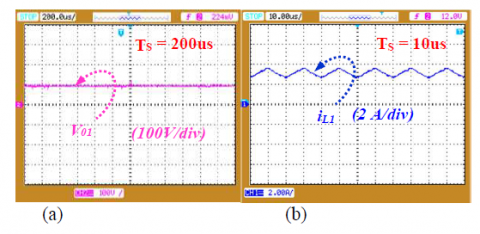## 9b.png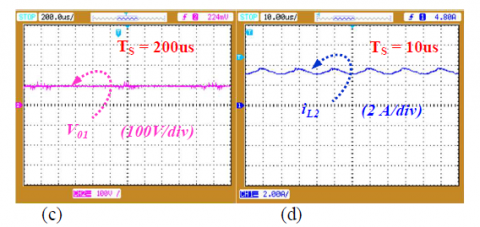Figure 9. Experimental results: (a) V01, (b) iL1) (c) V02), (d) iL2

## 10.png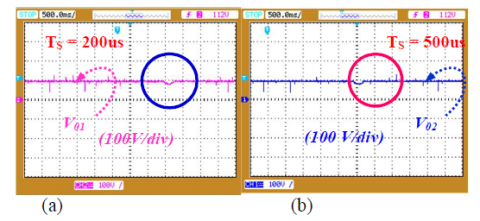Figure 10. Output voltage at load variations: (a) V01 (b) V02

## 11a.png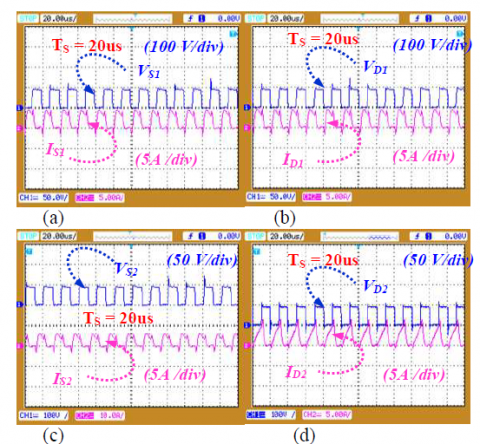## 11b.png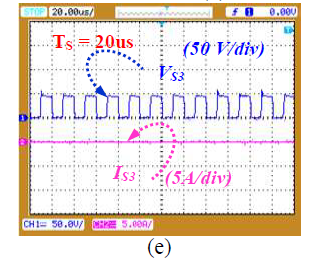Figure 11. Voltage & current stresses on: (a) S1, (b) D1, (c) S2 (d) S3, and (e) D2.

## 12a.png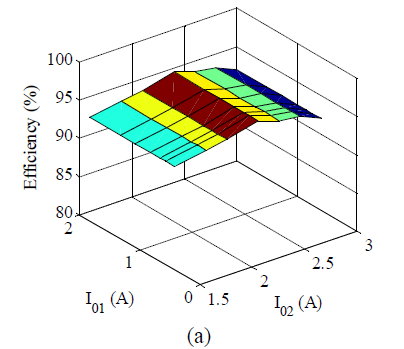## 12b.png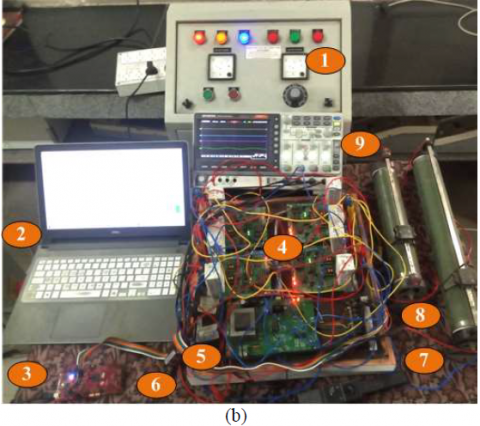Figure 12. (a) Proposed converter efficiency, (b) Experimental photograph developed in the laboratory: (1) Voltage source, (2) Laptop (3) DSP 28335 Controller, (4) IGBT power circuit module, (5) Inductors, (6) Capacitors, (7) Current probe, (8) Load (R1) and (R2), and (9) DSO.

7. Conclusion

A new structure of a SIDO converter is proposed in this paper. The configuration is free from operational constraints on duty ratio and inductor currents. This paper also describes the working principle and operating modes of the proposed configuration in detail. The proposed converter is helpful to operate both in boost and buck-boost modes to obtain a different set of output voltages. The results are illustrated that the cross-regulation issues are eliminated with this converter. Finally, all the stated features of the proposed converter have been well established with simulation and experimental verifications.

References

 Rehman, Z., Al-Bahadly, I., and Mukhopadhyay, S. (2015). Multi-input DC-DC converters in renewable energy applications – An overview. Journal of Renewable and Sustainable Energy Reviews, 41, 521– 539.

 Babaei, E. and Abbasi, O. (2016). Structure for multi- input multi-output dc-dc boost converter. IET Power Electronics, 9, (1), 9–19.

 Heris, P. C., Saadatizadeh, Z. and Ebrahim B (2018).A New Two Input-Single Output High Voltage Gain Converter with Ripple-Free Input Currents and Reduced Voltage on Semiconductors.IEEE Transactions on Power Electronics,15(2), 232-242.

 Amir, F., Mehdi A., Mehran S. (2018). Design, analysis, and implementation of a multi-port DC-DC converter for renewable energy applications, IET Power Electronics, 12(3), 465-475.

 Santanu, K. M., Khirod Kumar N., Mandeep Singh R. and VimalaDharmarajan J. (2019). Switched-Boost Action Based Multiport Converter, IEEE Transactions Babaei, E. and Abbasi, O. (2016). Structure for multi- input multi-output dc-dc boost converter. IET Power Electronics, 9, (1), 9–19.

Industrial Applications, 55(1), 964–975.

 Xiaomin L., Lakshmi VarahaIyer, K., Chunyan L., Kaushik M. and Narayan C. K. (2016). Design and Testing of a Multi-port Sustainable DC Fast-charging System for Electric Vehicles, Electric Power Components and Systems, 44(14), 1576–1587.

 Babaei, E and Abbasi, O. (2016). A new topology for bidirectional multi‐input multi‐output buck direct current–direct current converter, International Transactions Electric. Energy System, 27(2), 1–15.

 Patra, P., Patra, A and Misra N. (2012). A single-inductor multiple-output switcher with simultaneous buck, boost, and inverted outputs, IEEE Transactions on Power Electronics, 27(4), 1936–51.

 Abbasi, M., Afifi, A., and Pahlavani, M. R. A. (2018). Comments on “A single-inductor multiple-output switcher with simultaneous buck, boost, and inverted outputs, IEEE Transactions Power Electronics.

 Yi-Chieh, H., Jing-Yuan L., Chii-Hwa, W. and Sz-Wei C. (2020). An SIMO Step-Down Converter with Coupled Inductor”, In 2020 International Symposium on VLSI Design, Automation and Test (VLSI-DAT), Hsinchu, Taiwan.  2020, DOI: 10.1109/VLSI-DAT49148.2020.9196435.

 Gayatri N. and Shabari N. (2018). Comparing Performances of SIDO Buck Converters”, In 2018 IEEE International Conference on Power Electronics, Drives and Energy Systems (PEDES), Chennai, India, 2018.

 Yanqi, Z., Jianping G., and Nang L. K. (2020). A Single- Inductor Multiple-Output Buck/Boost DC-DC Converter With Duty-Cycle and Control-Current Predictor, IEEE Transactions Power Electronics, 35(11), 12022-12039.

 Xinan Z., Benfei W., Xiaojun T., Hoay B. G., Herbert H.- C., Tyrone F. (2020), Deadbeat Control for Single- Inductor Multiple-Output DC-DC Converter with Effectively Reduced Cross Regulation, IEEE Journal of Emerging and Selected Topics in Power Electron, 8(4), 3372 – 3381.

 Dasika, J. D., Bahrani, B., Saeedifard, M., Karimi, A. and Rufer, A. Multivariable control of single-inductor dual- output buck converters, IEEE Transactions Power Electronics, 29(4), 2061-2070.

 Eladio D., Salvador P. Litrán, and María B. F. (2020). Configurations of DC-DC converters of one input and multiple outputs without transformer, IET Power Electronics, 13(12), 2658-2670.

 Santos, E. C. D. (2013). Dual-output dc-dc buck converters with bidirectional and unidirectional characteristics, IET Power Electron.ics 6(5), 999–1009.

 Ray, O, and Josyula, A, Mishra, S. and Joshi, A. (2015). Integrated dual-output converter, IEEE Transactions on Industrial Electronics, 62(1), 371-382

 Chen, G, Deng, Y, Dong, J, Hu, Y., Jiang, L, and He, X. (2017). Integrated multiple-output synchronous buck converter for electric vehicle power supply, IEEE Transactions on Vehicular Technology, 66(7), 5752- 5761.

 Ranjana, M. S. B., Reddy, N. S. and Kumar, R. K. P. (2014). A novel sepic based dual output dc-dc converter for solar applications, In 2014 Power and Energy Systems: Towards Sustainable Energy Conference (PESTSE 2014), 1-5

 Marjani, J., Imani, A., Afjei E., and Hekmati, A. (2016). A new dual output dc-dc converter with enhancing output voltage level, In 24th Iranian Conference on Electrical Engineering (ICEE), 573-577.

 Chen, G., Jin, Z., Deng, Y., He, X. and Qing, X. (2018). Principle and Topology Synthesis of Integrated Single- Input Dual-Output and Dual-Input Single-Output DC-DC Converters, IEEE Transactions on Industrial Electronics, 65(5), 3815-3825.

 Zahra S., Pedram C. H., Ebrahim B. and Mehran S. (2019). A New Non-Isolated Single-Input Three-Output High Voltage Gain Converter with Low Voltage Stresses on Switches and Diodes, IEEE Transactions on Industrial Electronics, 66(6), 4308-4318.

 Ganjavi, A., Ghoreishy, H. and Ahmad, A. A. (2018). A novel single-input dual-output three-level dc-dc converter, IEEE Transactions on Industrial Electronics, 65(10), 8101–8111.

 Ahsanuzzaman, S. M. Prodic, A., and Johns, D. A. (2016). An integrated high density power management solution for portable applications based on a multi-output Switched-Capacitor Circuit,” IEEE Transactions on Power Electronics, 31(6), 4305-4323.

Fan, L., & Ma, X. (2022). Maximum power point tracking of PEMFC based on hybrid artificial bee colony algorithm with fuzzy control. Scientific Reports, 12(1), 1-12.

Karthikeyan, B., Sundararaju, K., & Palanisamy, R. (2021). ANN-Based MPPT Controller for PEM Fuel Cell Energized Interleaved Resonant PWM High Step Up DC-DC Converter with SVPWM Inverter Fed Induction Motor Drive. Iranian Journal of Science and Technology, Transactions of Electrical Engineering, 45(3), 861-877.

Srinivasan, S., Tiwari, R., Krishnamoorthy, M., Lalitha, M. P., & Raj, K. K. (2021). Neural network based MPPT control with reconfigured quadratic boost converter for fuel cell application. International Journal of Hydrogen Energy, 46(9), 6709-6719.

Premkumar, K., Vishnupriya, M., Thamizhselvan, T., Sanjeevikumar, P., & Manikandan, B. V. (2021). PSO optimized PI controlled DC‐DC buck converter‐based proton‐exchange membrane fuel cell emulator for testing of MPPT algorithm and battery charger controller. International Transactions on Electrical Energy Systems, 31(2), e12754.

 Ramanarayanan, V. (2008). Course Material on Switched Mode Power Conversion, IISc., Bangalore.

 Kazimierczuk,M. K. (2008). Pulse-Width Modulated DC–DC Power Converters, Wiley Press, 1stedn.

 Alishah, R. S., Nazarpour, D., Hosseini, S. H. and Sabahi, M., 2015. Reduction of power electronic elements in multilevel converters using a new cascade structure, IEEE Transactions on Industrial Electronics, 62(1), 256– 269.

 Pera, M. C., Candusso, D., Hissel, D., & Kauffmann, J. M. (2007). Power generation by fuel cells. IEEE Industrial Electronics Magazine, 1(3), 28-37.